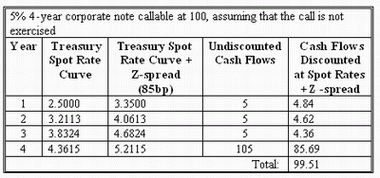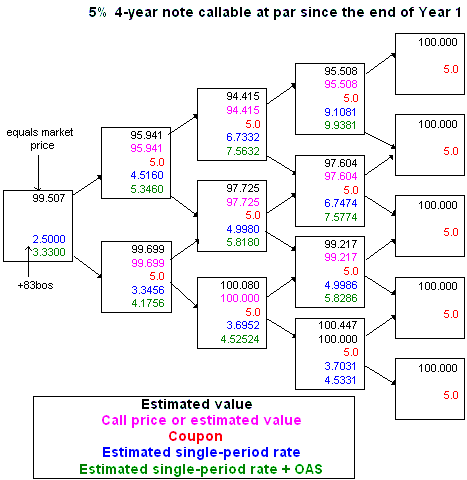### Why should I choose AnalystNotes?

Simply put: AnalystNotes offers the best value and the best product available to help you pass your exams.

Yield spread is the difference in yields between a target bond and a benchmark bond. They typically have the same maturity but different credit quality. Treasury securities are usually used as the benchmark bonds.

The nominal spread is the difference between the yield for a non-Treasury bond and a comparable-maturity Treasury coupon security. It is the traditional yield spread measure. For example, suppose a 10 year, 8%-coupon bond is selling at \$104.19, yielding 7.40%. The 10-year Treasury bond (6% coupon rate) has a YTM of 6.00%. Therefore, the nominal spread is 7.40% - 6.00% = 1.40%, or 140 basis points.

The nominal spread has two limitations:

• For both bonds, it fails to consider the term structure of the spot rates. YTM is a single interest rate only.
• For bonds with embedded options, future interest rate volatility may alter the cash flows of the non-Treasury bond.

Let's assume that the interest rate tree (based on 15% annual volatility assumption) that we used in our earlier examples is based on the Treasury spot rate curve. A 5% 4-year corporate note is trading at 99.5 and is callable at par since the end of year 1. We are asked to determine the spread over the Treasury curve at which the note is trading.

There are two alternative definitions of a spread.

The Z-spread (zero-volatility spread) is the interest rate premium that, when added to all spot rates on the Treasury curve (or a forward rate curve), will make the bond's current value equal to its market price. A trial-and-error procedure is required to determine the Z-spread: we simply iterate different values of this spread until the discounted value becomes equal to the market price of the bond. It is a measure of the spread that the investor would realize over the entire Treasury spot rate curve if the bond is held to maturity.

In our case, Z-spread is just above 85 bps, as shown in the table below:This spread compensates investors for three types of risk: liquidity risk, credit risk and option risk. In order to compare callable bonds with non-callable instruments, we need a spread measure that does not include compensation for option risk. Otherwise, the Z-spread of callable bonds would always be higher than that of non-callable bonds with similar liquidity and credit risks, but the higher spread would not indicate which of the two bonds has a greater value. For that reason, we use the spread measure that is adjusted for option risk, the option-adjusted spread (OAS).

OAS is the interest rate premium that, when added to all one-period rates on the tree, gives the market value of the note at the root. It is the spread adjusted for option risk.

The following exhibit demonstrates that OAS equals 83 basis points in our case. We add this premium to each one-year rate on the tree and value the note using the usual backward discounting approach, making nodal decisions whether the call is exercised at each node.CFA Institute often asks for interpretation of OAS and comparison of this spread with Z-spread. It is important to remember that OAS is the compensation for difference in credit and liquidity risks between the valued instrument and the benchmark interest rates. The reason why the spread is "option-adjusted" is because on the potential paths the cash flows are adjusted to reflect the options embedded in the bond.

• If the benchmark interest rates are derived from on-the-run Treasury curve, OAS is compensation for the issuer's liquidity risk and credit risk.
• If the benchmark rates are based on the issuer's on-the-run curve, OAS compensates for additional liquidity risk of the notes only.
• What OAS does not compensate for is the option risk, because this risk was already recognized in our nodal decisions on the interest rate tree.

Consequently, OAS is a more appropriate measure of spread when comparing issues with embedded options. The analyst then asks: Is the OAS great enough to compensate for credit and liquidity risk? All else equal, we should buy large OAS bonds.

In our case, the difference between Z-spread and OAS is 85 - 83 = 2 bps, which is the cost of the call option. The following formula is often tested on the exam:

Z-spread = OAS + Option cost

The option cost is measured in this way because if rates do not change, the investor would earn the Z-spread.

User Comment
novelt Review this section again...note the items that are likely on the exam!
dblueroom OAS is a certainty on the exam!
vi2009 Z spread = OAS + Option cost
i.e. Z-spread covers for option, liquidity and credit risk.
OAS is option adjusted, it only covers for liquidity risk and credit risk if both are appropriate.
shihu Question: An investor purchases a bond that is putable at the option of the holder. The option has value. He has calculated the Z-spread as 223 basis points. The options adjusted spread will be:

Answer: greater than 223 basis points

I dont understand if OAS = z Spread - Option Cost., and the option has value to the investor, why would it be greater (instead of smaller).

If anyone can further expand on this, and the below LOS in particular, id appreciate it. I guess I just don't understand the concept behind it.

For callable bonds, zspread > OAS and option cost > 0
Forr putable bonds, z spread < OAS and option cost < 0
jmorris For putable bonds, zspread < OAS and option cost <0
option cost < 0 means that option cost is negative which implies that
OAS = Zspread - (-option cost)
OAS = Zspread + option cost

To explain this. Option has a premium named as option cost. For a callable bond the bond holder (investor) is the option writer and he has received premium which is equal to the option cost. The borrower or bond issuer in this case is the buyer of the option and he has paid the option premium (cost) by issuing the bond at a price less than the price of an option free bond. For this particular reason

Price of Callable bond = Price of Option free bond - Option Cost

Conversly for a putable bond the price of the bond is higher than an identical option free bond because the option writer in this regard is the Bond Issuer and the buyer of the bond is the Bond Holder. The Buyer purcahses the bond and also the option which gives him the right of returning the bond at the price higher than the market price.

Price of a Putable bond = Price of Option free bond + Option Cost
jmorris Apologies for the wordy response, wanted to make the explanation as explicit as possible:

A call option on a bond is an option for the issuer, written by the bondholder. It gives the issuer the option to buy redeem the bonds prior to maturity. The bondholder receives payment for giving this option to the issuer, therefore Option Cost > 0.

A Put Option is an option for the bondholder, written by the issuer. It gives the bondholder the option to demand early repayment of principle at the exercise date(s). The bondholder makes a payment for having this option from the issuer, therefore Option Cost < 0.

Note these "payments" are not separate, rather they are priced into the coupon.

So these Option Cost values are both from the perspective of the bondholder and from that perspective make complete sense.

Now for this statement:
OAS = Z-Spread - Option Cost
or
Option Cost = Z-Spread - OAS

Remember the Z-Spread alone includes the effect of embedded options, so think what effect you'd expect embedded options to have on yields.

Callable Bond - Increase yields (compared to identical option-free bond), to compensate bondholder for the fact issuer can decide to redeem bonds prior to maturity.

Puttable Bond - Decreased yields, to "penalise" bondholder for the fact he/she can demand repayment of principle prior to maturity.

Now think about what the OAS is doing, it's removing the effect of the embedded option

On the callable bonds OAS < Z- Spread:
The Call Option increases the yield (+ve), so when we remove the effect of this option to get the OAS, it will be less than the Z-Spread (which included that effect).

On the puttable bonds OAS > Z- spread:
The Put Option decreases the yield (-ve), so when we remove the effection of this option to get the OAS, it will be greater than the Z-Spread (which included that effect).
Rivermax Useful explanations thank you jmorris.
zzhumanov Z-Spread = Credit risk + Liquidity risk + Option risk (or cost);
OAS = Credit risk + Liquidity risk

For callable bonds: Option cost > 0 (as explained above) ==> OAS < Z-spread
For putable bonds: Option cost < 0 =================> OAS > Z-spread